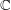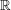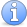## Complex analysis front page

### Quick description

Complex analysis is the study of complex-differentiable functions. In a first course on complex analysis, these functions are normally defined on domains, that is, connected open subsets of. The definition of a complex-differentiable function looks just the same as the definition of a differentiable function fromto, but this appearance is misleading: complex-differentiable functions are so unlike real-differentiable functions that they are given a different name. They are known as holomorphic functions. Holomorphic functions can also be defined on Riemann surfaces and complex manifolds. At a more advanced level, there are many connections between complex analysis and geometry. More generally, complex analysis is a tool that is used throughout mathematics.

### Prerequisites

A basic knowledge of the main theorems of complex analysis.Attention This article is in need of attention. This is a hastily-put-together page in order to give a parent to the few articles on complex-analysis tricks we have so far.

At the moment there are very few complex analysis articles on the Tricki, so here is a list of all of them. Later, it will almost certainly be necessary to add another level to the hierarchy. In fact, a start has been made on that process, by grouping together two articles into a general "contour integration" heading.

To calculate an integral round a closed path, use theorems rather than direct calculation

Contour integration tricks

Proving that functions are holomorphic

Properties of holomorphic functions

Conformal maps

Singularities